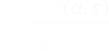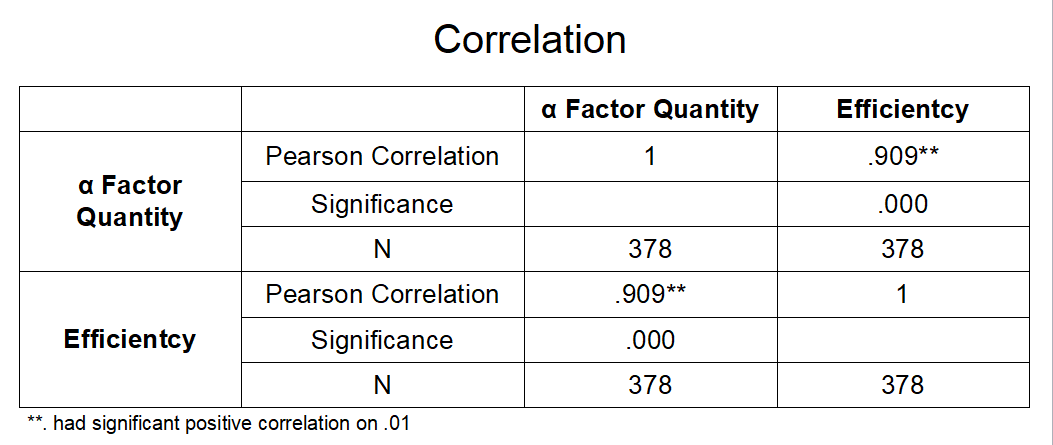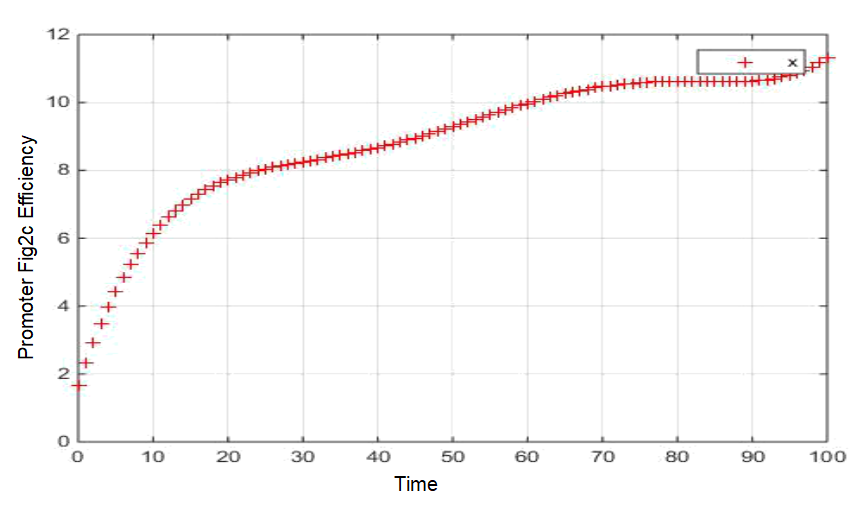Model 1

# intorduction

Based on the procedure of our case, we hypothesized that the duration of induced spy yeast ( the information carrying yeast, designated as "spy yeast" ) death was relevant to the initial amounts of two types of yeast and production of secreted α factor. The duration of spy yeast death was proportional to the expression of Bax gene, while Bax gene expression depended on the activation efficiency of the promoter Fig2c. Thus, we should eventually determine whether the transcription efficiency of the Fig2c promoter is directly relevant to these three parameters. To verify the hypothesis, we used correlation analysis to calculate the correlation
<>coefficients.
Coefficients is :The data obtained from the wet laboratory, starting efficiency and initial alpha dosage of promoter Fig2c, were calculated correlation coefficient and fitted into a curve below.

# RESULTS

The result is as followed:

Result 1: Correlation Coefficient of efficiency promoter Fig2c and initial dosage of killing yeast is below, and we find out their coefficient have strong correlation which verify our hypothesize is right.Result 2: Fitting into curve of two correlation coefficient.